Get instant live expert help with Excel or Google Sheets“My Excelchat expert helped me in less than 20 minutes, saving me what would have been 5 hours of work!”

#### Post your problem and you'll get expert help in seconds

Your message must be at least 40 characters
Our professional experts are available now. Your privacy is guaranteed.

# How to Sort Text and Number with a Formula

We can sequentially arrange text and numbers in excel based on ranks assigned to the text and numbers in another cell. We can do this with the COUNTIF, ISTEXT, INDEX, and MATCH Functions. This step by step tutorial will assist all levels of Excel users in sorting text and numbers using a formula.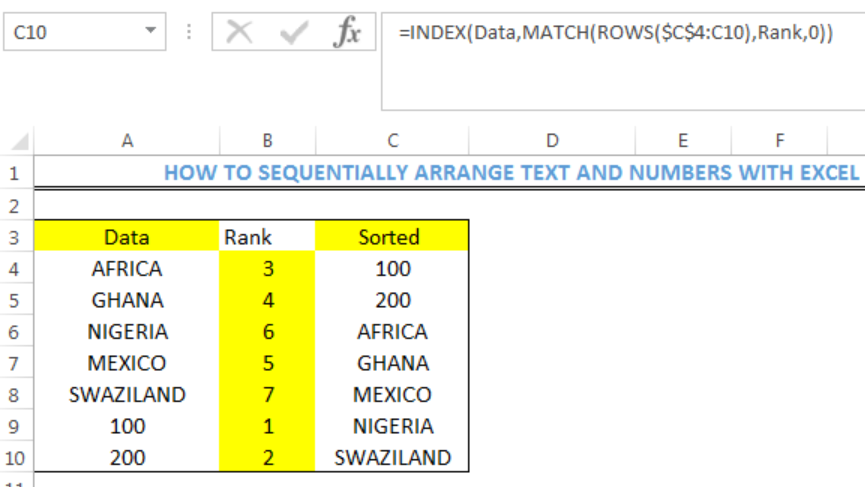Figure 1: Result of Sequentially Sorted Data

## Formula

Ranking formula: `=COUNTIF(Data,"<="&A4)+(COUNT(Data)*ISTEXT(A4))`

Sorting formula: `=INDEX(Data,MATCH(ROWS(\$C\$4:C4),Rank,0))`

## Setting up the Data

• We will input the numeric and text values into the cells as shown in figure 2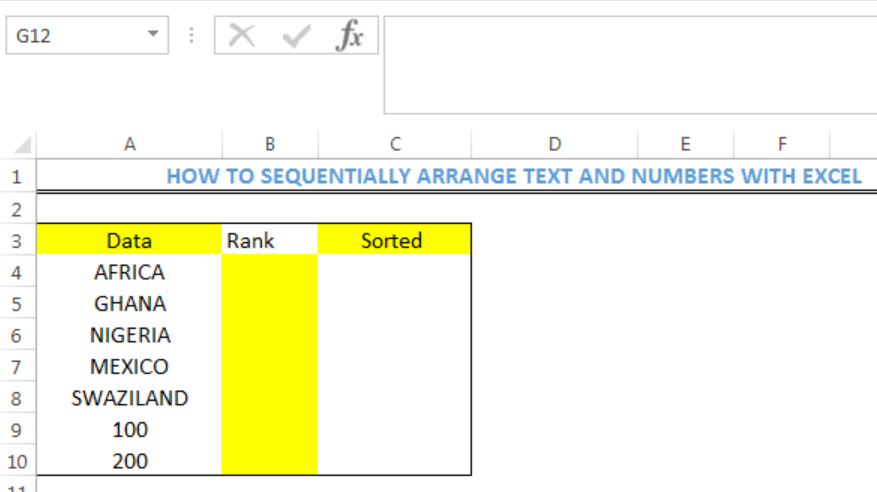Figure 2: Setting up the Data

• We must note that Data and Rank are named ranges. This means that Cell A4 to Cell A10 is collectively named as Data and Cell B4 to Cell B10 is collectively named as Rank. To do this, highlight Cell A4 to Cell A10 and go to the position of G12 (REFER TO FIGURE 2) on your spreadsheet. Click on it and name it as Data. Ensure to press the enter key. Do the same for Rank.

## Ranking the Data

• We will click on Cell B4
• We will insert the formula and press enter: `=COUNTIF(Data,"<="&A4)+(COUNT(Data)*ISTEXT(A4))`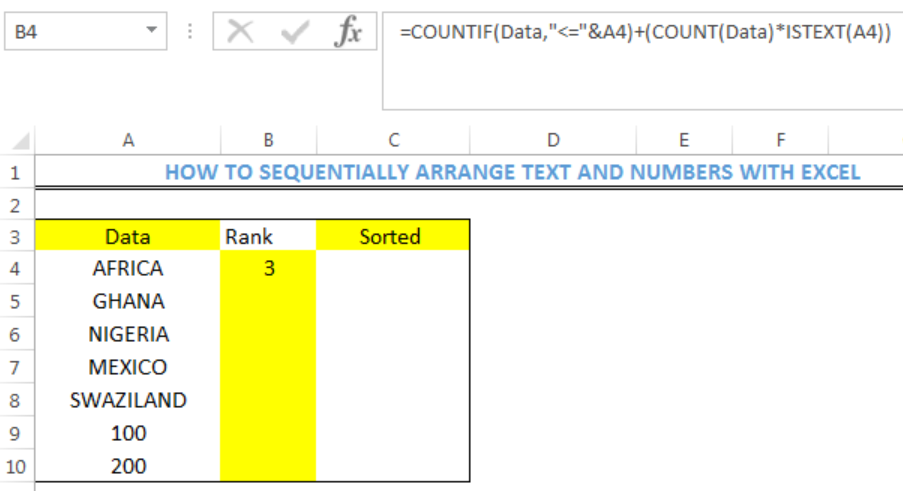Figure 3: Ranking the Data

• We will click on the fill handle and drag down to copy the formula into the other cells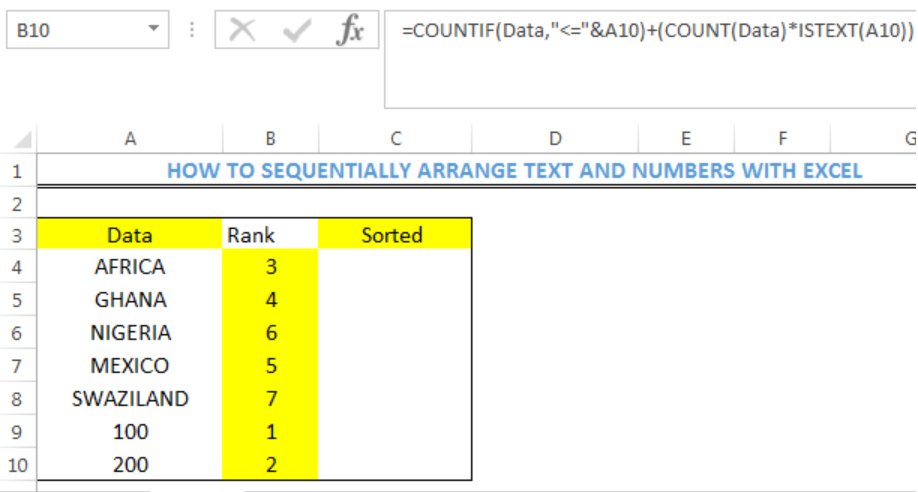Figure 4: Ranked Data

## Sorting the Data Sequentially

• We will click on Cell C4
• We will insert the formula and press enter: `=INDEX(Data,MATCH(ROWS(\$C\$4:C4),Rank,0))`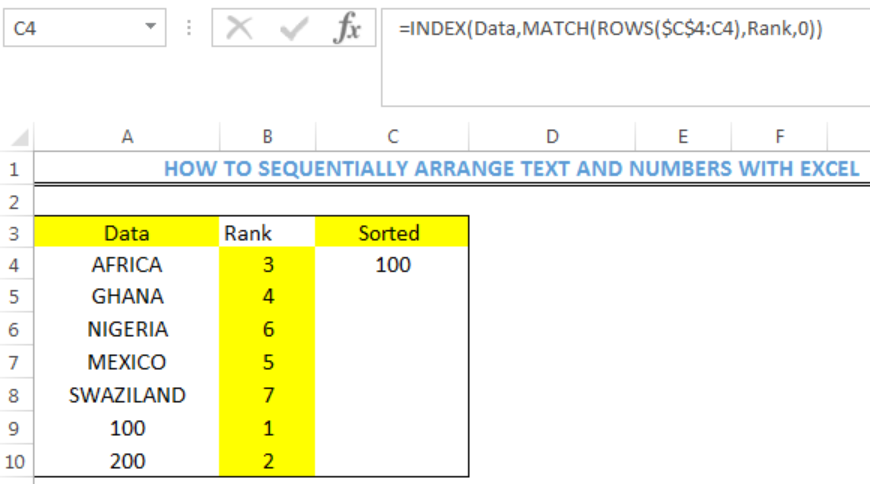Figure 5: Sorting the Data Sequentially

• We will click on the fill handle and drag down to copy the formula into the other cells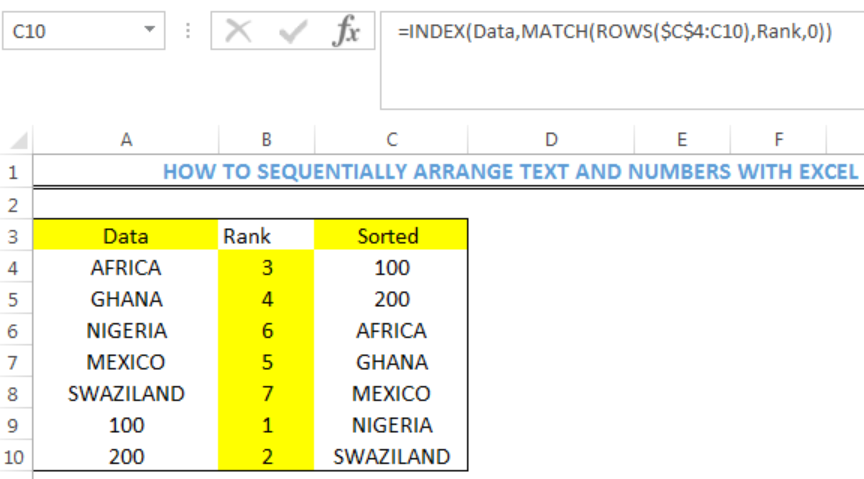Figure 6: Result of Sequentially Sorted Data

## Explanation

When ranking the data, the COUNTIF section of the formula is suitable for ranking only all text values or all numeric values. To accommodate a count of the numeric values into a single formula, the COUNT FUNCTION is used.

The COUNT FUNCTION gets the COUNT of numeric values in the data. The value is then multiplied by the logical result of ISTEXT. ISTEXT detects if the value is a text or number and returns TRUE or FALSE.

## Handling Blank Cells

Assuming Cell A7 is a blank cell, we can still rank the values with the formula discussed above. However, zero will be the assigned rank to the empty cell. To ensure that this zero does not interfere with the sorted values, we will insert the formula below into Cell C4, PRESS ENTER and use the fill handle to copy the formula into the remaining cells.

`=IFERROR(INDEX(Data,MATCH(ROWS(\$C\$4:C4),Rank,0)),"")`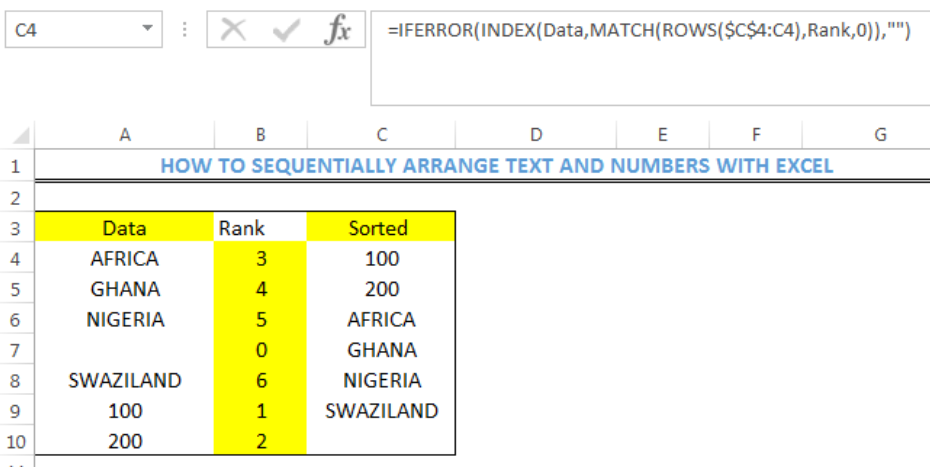Figure 7: Handling Blank Cells

## Note

• To sort data that contains duplicate values, use the formula below TO RANK THE DATA and adhere to the aforementioned steps TO SORT THE DATA

`=COUNTIF(Data,"<"&A4)+(COUNT(Data)*ISTEXT(A4))+COUNTIF(\$A\$4:A4,A4)`

## Instant Connection to an Expert through our Excelchat Service

Most of the time, the problem you will need to solve will be more complex than a simple application of a formula or function. If you want to save hours of research and frustration, try our live Excelchat service! Our Excel Experts are available 24/7 to answer any Excel question you may have. We guarantee a connection within 30 seconds and a customized solution within 20 minutes.

### Did this post not answer your question? Get a solution from connecting with the expert.Another blog reader asked this question today on Excelchat:
Related blogs## Subscribe to Excelchat.coAnother blog reader asked this question today on Excelchat: